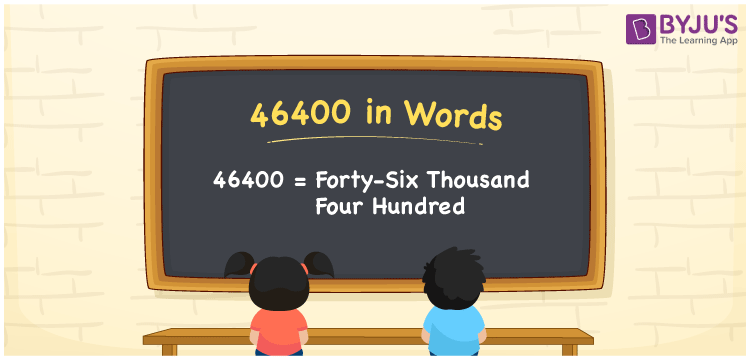# 46400 in Words

46400 in words is “forty-six thousand four hundred”. The conversion from 46400 into words can be performed using the place value system. If you invested Rs. 46400 on purchasing a laptop, you can write it as “I invested Rs. Forty-six thousand four hundred on purchasing a laptop”. Also, 46400 is a cardinal number

 46400 in Words: Forty-six Thousand Four Hundred. Forty-six Thousand Four Hundred in Numerical Form: 46400.

## 46400 in English Words## How to Write 46400 in Words?

Learn the place values of 46400 using the below place value table.

 Ten-thousands Thousands Hundreds Tens Ones 4 6 4 0 0

The expanded form of 46400 is as follows:

= 4 × Ten thousand + 6 × Thousand + 4 × Hundred + 0 × Ten + 0 × One

= 4 × 10000 + 6 × 1000 + 4 × 100 + 0 × 10 + 0 × 1

= 40000 + 6000 + 400

= 46400

= Forty-six thousand four hundred

Hence, 46400 in words is forty-six thousand four hundred.

46400 in words – Forty-six thousand four hundred

Is 46400 an odd number? – No

Is 46400 an even number? – Yes

Is 46400 a perfect square number? – No

Is 46400 a perfect cube number? – No

Is 46400 a prime number? – No

Is 46400 a composite number? – Yes

## Frequently Asked Questions on 46400 in Words

Q1

### How to write 46400 in words?

46400 in words is forty-six thousand four hundred.

Q2

### Simplify 46000 + 400, and express it in words.

Simplifying 46000 + 400, we get 46400. Hence, 46400 in words is forty-six thousand four hundred.

Q3

### Is 46400 a composite number?

Yes, 46400 is a composite number.